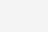# Python海象运算符的使用1、在判断条件下允许操作。在一定程度上简化了代码，但降低了可读性。

```i = len((l := [1, 2, 3]))  # 先对l进行赋值，在对i赋值
while (i := i - 1) >= 0:  # 允许在表达式中运算
print(l[i], end=' ')```

2、python中的海象操作符较少使用，允许在判断中进行简单的赋值操作，没有其他特殊意义，只需了解其存在即可。

```l = [1, 2, 3]
i = len(l) - 1
while i >= 0:
print(l[i], end=' ')
i -= 1```